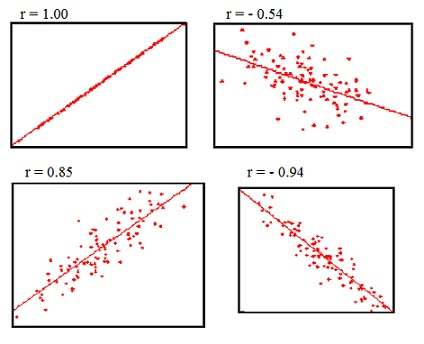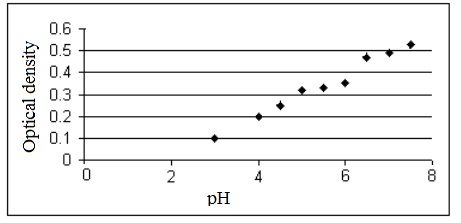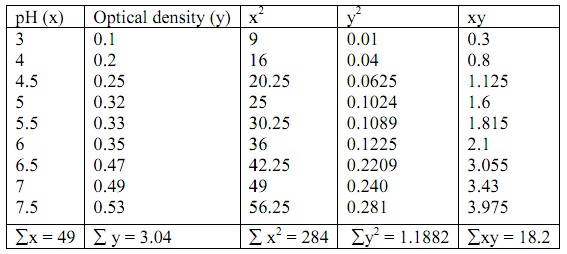#### Correlation and Regression, Chemistry tutorial

Introduction:

The Pearson Product-Moment Correlation Coefficient (r), or in short correlation coefficient is a measure of the degree of linear relationship between the two variables, generally labeled X and Y. whereas in regression the emphasis is on predicting one variable from the other, in correlation the emphasis is on the degree to which a linear model might illustrate the relationship between the two variables. In regression the interest is directional, one variable is predicted and the other is the predictor; in correlation the interest is non-directional, the relationship is the vital feature.

Product-Moment Correlation:

A general process of estimating how well the experimental points fit a straight line is to compute the product moment correlation.

Definition:

Pearson's correlation coefficient between the two variables is stated as the covariance of the two variables divided via the product of their standard deviations:

ρX,Y = cov (X,Y)/σXσY = E[(X - μX)(Y - μY)]/σXσY

The formula above states the population correlation coefficient, generally symbolized by the Greek letter ρ (rho). Substituting estimates of the covariance and variances based on the sample provides the sample correlation coefficient, generally represented by r:

r = Σ{(x1-x‾)(y1-y‾)}/[{(x1-x‾)2}{(y1-y‾)2]

The correlation coefficient might take on any value between plus and minus one.

-1.00 ≤ r ≤ +1.00

The sign of correlation coefficient (+, -) states the direction of the relationship, either positive or negative. The positive correlation coefficient signifies that as the value of one variable rises, the value of the other variable rises; as one reduces the other reduces. A negative correlation coefficient points out that as one variable rises, the other reduces, and vice-versa.

Considering the absolute value of the correlation coefficient measures the strength of the relationship. The correlation coefficient of r = .50 points out a stronger degree of linear relationship than one of r =.40. Similarly a correlation coefficient of r = -.50 illustrates a greater degree of relationship than one of r = .40. Therefore a correlation coefficient of zero (r = 0.0) points out the absence of a linear relationship and correlation coefficients of r = +1.0 and r = -1.0 point out a perfect linear relationship.

Understanding and Interpreting the Correlation Coefficient:

The correlation coefficient might be comprehended by different means, each of which will now be examined in turn.

The scatter plots represented below possibly best describe how the correlation coefficient changes as the linear relationship between the two variables is modified. If r = 0.0 the points scatter broadly regarding the plot, the majority falling roughly in the shape of a circle. As the linear relationship rises, the circle becomes more and more elliptical in shape till the limiting case is reached (r = 1.00 or r = -1.00) and all the points fall on the straight line.

A number of scatter-plots and their related correlation coefficients are represented below in order that the student might better estimate the value of the correlation coefficient based on the scatter-plot in the related computer exercise.Fig: Slope of the Regression Line of z-scores

The correlation coefficient is the slope (b) of the regression line whenever both X and Y variables have been transformed to z-scores. The bigger the size of the correlation coefficient, the steeper the slope. This is associated to the difference between the intuitive regression line and the actual regression line illustrated above.

This interpretation of the correlation coefficient is possibly best described by an illustration comprising numbers.

Variance Interpretation:

The squared correlation coefficient (r2) is the proportion of variance in Y which can be accounted for by knowing X. On the contrary, it is the proportion of variance in X which can be accounted for by knowing Y.

One of the most significant properties of variance is that it might be partitioned into separate additive parts. For illustration, consider shoe size. The theoretical distribution of shoe size might be represented as shown:Fig: Theoretical distribution of shoe

Whenever the scores in this distribution were partitioned into two groups, one for males and one for females, the distributions could be symbolized as above:

Whenever one knows the sex of an individual, one knows something regarding that person's shoe size, as the shoe sizes of males are on the average somewhat bigger than females. The variance in each distribution, male and female, is variance that can't be predicted on the basis of sex or error variance, as whenever one knows the sex of an individual, one doesn't know precisely what that person's shoe size will be.

Instead of having just two levels, the X variable will generally encompass numerous levels. The preceding argument might be extended to encompass this condition. This can be illustrated that the total variance is the sum of the variance which can be predicted and the error variance, or variance which can't be predicted. This relationship is concluded below:

S2Total = S2Predicted + S2Error

S2Predicted = S2Total - S2Error

This formula might be represented in terms of the error variance, instead of the predicted variance as:

r2 = (S2Total - S2Error)/S2Total

r2 = (S2Total/S2Total) - (S2Error/S2Total)

r2 = 1 - (S2Error/S2Total)

The error variance, s2ERROR, is predicted by the standard error of estimate squared, S2Y,X. The total variance (S2TOTAL) is simply the variance of YS2Y. The formula now becomes:

r2 = 1 - S2YX/S2Y

Resolving for sY.X, and adding a correction factor (N-1)/(N-2), results the computational formula for the standard error of estimate,

SYX = √[(N-1)/(N-2)] S2Y (1-r2)

This holds the vital relationship between the correlation coefficient, the variance of Y, and the standard error of estimate. Since the standard error of approximation becomes large relative to the total variance, the correlation coefficient becomes smaller. Therefore the correlation coefficient is a function of both the standard error of estimate and the total variance of Y. The standard error of estimate is the absolute measure of amount of error in prediction, whereas the correlation coefficient squared is a relative measure, relative to the total variance.

Further Calculation of the Correlation Coefficient:

The simplest process of computing a correlation coefficient is to make use of a statistical calculator or computer program. Barring that, the correlation coefficient might be calculated by using the given formula:

r = [i=1ΣN ZXZY]/(N-1)

Regression Analysis:

In statistics, the regression analysis comprises many methods for modeling and examining several variables, whenever the focus is on the relationship between the dependent variable and one or more independent variables. More particularly, regression analysis assists one to understand how the typical value of the dependent variable modifies whenever any one of the independent variables is varied, whereas the other independent variables are held fixed. Most generally, regression analysis approximates the conditional expectation of the dependent variable provided the independent variables - that is, the average value of the dependent variable if the independent variables are held fixed. In regression analysis, it is as well of interest to characterize the variation of the dependent variable around the regression function that can be illustrated via a probability distribution.

Regression analysis is broadly employed for prediction and forecasting, where its use consists of the substantial overlap by the field of machine learning. Regression analysis is as well employed to comprehend which among the independent variables are associated to the dependent variable, and to explore the forms of such relationships. In limited conditions, regression analysis can be employed to deduce causal relationships between the independent and dependent variables. Though this can lead to the illusions or false relationships, therefore caution is advisable: observe correlation doesn't imply causation. A large body of methods for carrying out regression analysis has been developed. Well-known processes like linear regression and ordinary least squares regression are parametric, in that the regression function is stated in terms of a finite number of unknown parameters which are estimated from the data. Nonparametric regression refers to methods which allow the regression function to lie in a particular set of functions that might be infinite-dimensional.

Regression analysis: fitting a line to the data

This would be tempting to attempt to fit a line to the data we have just analyzed - producing an equation which exhibits the relationship, in such a way that we might predict the body weight of mice by measuring their length, or vice-versa. The process for this is termed as linear regression.

Though, this is not strictly valid as linear regression is mainly based on a number of suppositions. In specific, one of the variables should be 'fixed' experimentally and/or exactly measureable. Therefore, the simple linear regression processes can be employed only whenever we define some of the experimental variable (temperature, pH, dosage and so on) and test the response of the other variable to it.

The variable which we fix (or select deliberately) is known as the independent variable. This is for all time plotted on the X-axis. The other variable is known as the dependent variable and is plotted on the Y-axis.

Assume that we had the given results from an experiment in which we measured the growth of a cell culture (as optical density) at various pH levels.

pH                        3     4       4.5     5       5.5       6     6.5     7     7.5

Optical density    0.1   0.2    0.25   0.32   0.33    0.35  0.47  0.49  0.53

We plot such results (figure below) and they propose a straight-line relationshipFig: straight-line relationship

By using the similar processes as for correlation set out a table as follows and compute Σx, Σy, Σx2, Σy2, Σxy, and (mean of y).

Table: Procedures for correlationNow compute, Σdx2 = Σx2 - (Σx)2/n = 17.22 in our case.

Compute Σdxdy = Σxy - ΣxΣy/n (this can be positive or negative) = +1.649

Now we wish for to use regression analysis to determine the line of best fit to the data. We have done almost all the work for this in the computation above.

The regression equation for y on x is: y = bx + a

Here, 'b' is the slope and 'a' is the intercept (that is, the point where the line crosses the y-axis)

We compute b as:

b = Σdxdy/Σdx2

= 1.649 x 17.22 = 0.0958 in our case

We compute 'a' as:

a = y‾ - bx‾

From the recognized values of y‾(0.3378), x‾(5.444) and b (0.0958) we therefore determine a (-0.1837). Therefore the equation for the line of best fit is: y = 0.096x - 0.184 (to 3 decimal places). To draw the line via the data points, we replace in this equation. For illustration:

If x = 4, y = 0.384, therefore one point on the line consists of the x, y coordinates (4, 0.384);

If x = 7, y = 0.488, therefore the other point on the line consists of the x, y coordinates (7, 0.488).

It is as well true that the line of best fit always passes via the point having coordinates x, y therefore we in reality need only one other computed point in order to draw a straight line.

Tutorsglobe: A way to secure high grade in your curriculum (Online Tutoring)